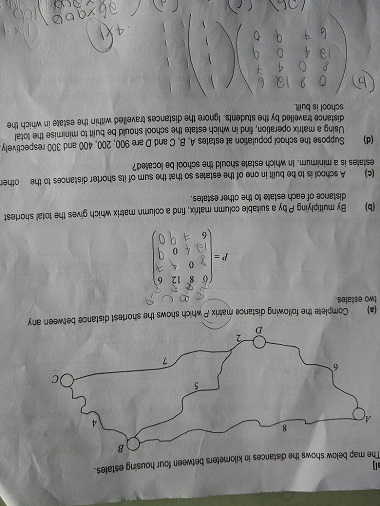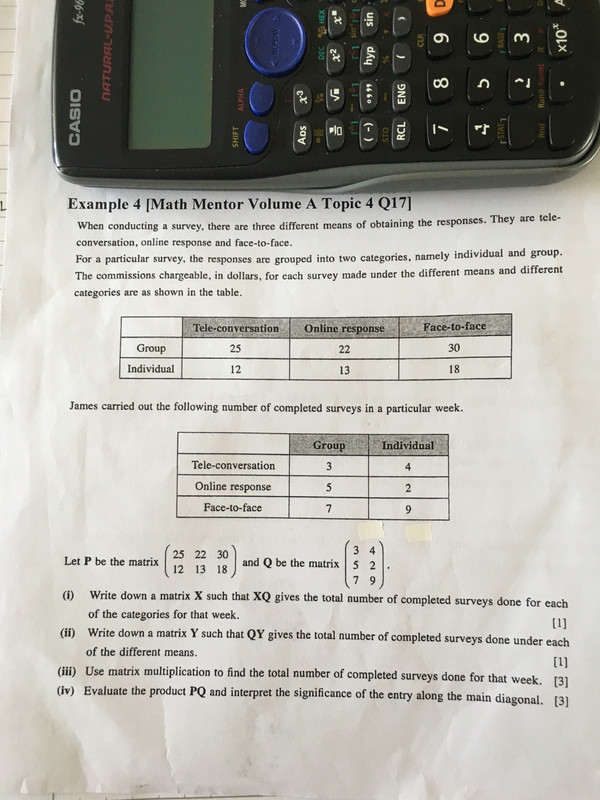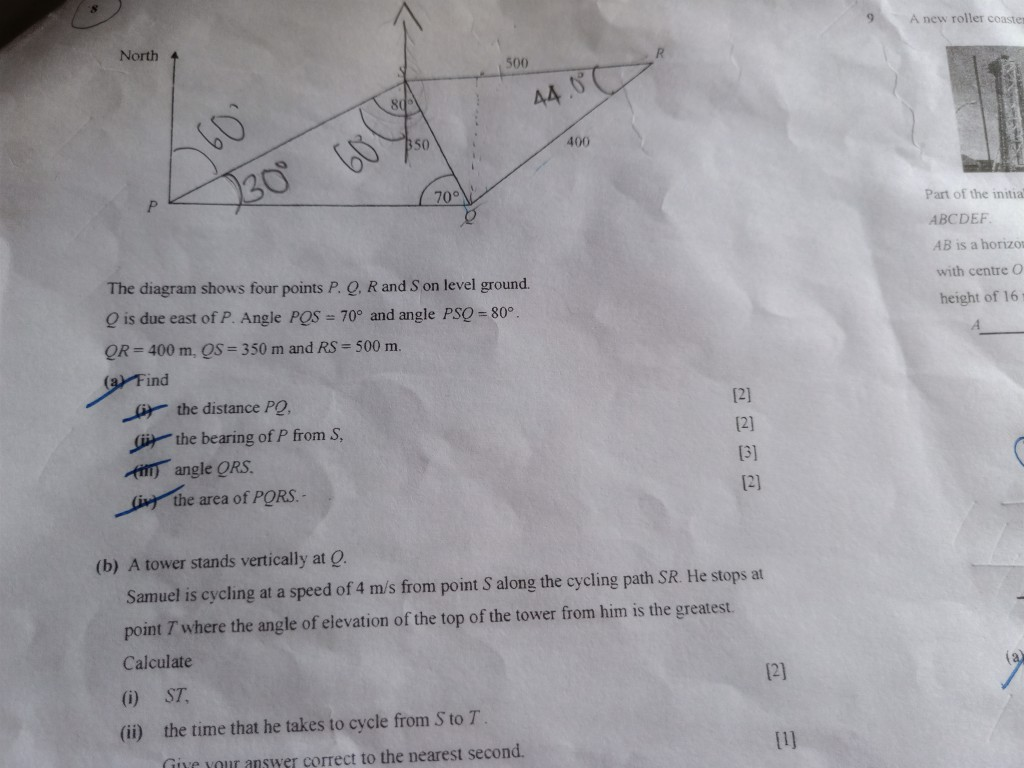## O-Level Elementary Math

PSLE marks the graduation of Primary school students and their entry into Secondary schools as teenagers. Discuss all issues about Secondary schooling here.
0rchid123
KiasuNewbiePosts: 3
Joined: Mon Sep 24,

### Re: O-Level Elementary Math

When f(x) is divided by x-1 and x+2 the remainders are 4 and -2 respectively. Hence find the remainder when f(x) is divided by X^2+X-2

0rchid123
KiasuNewbiePosts: 3
Joined: Mon Sep 24,

### Re: O-Level Elementary Math

When f(x) is divided by x-1 and x+2 the remainders are 4 and -2 respectively. Hence find the remainder when f(x) is divided by X^2+X-2

sundarshoba
KiasuNewbiePosts: 9
Joined: Fri Mar 30,

### Maths Question

Thanks

[img][img]https://i.postimg.cc/2SFdz5qJ/Mat1.jpg[/img][/img]

sundarshoba
KiasuNewbiePosts: 9
Joined: Fri Mar 30,

### Re: O-Level Elementary Math

ThanksalwaysLovely
YellowBeltPosts: 19
Joined: Wed Jan 14,

### Re: O-Level Elementary Math

When f(x) is divided by x-1 and x+2 the remainders are 4 and -2 respectively. Hence find the remainder when f(x) is divided by X^2+X-2
This is classified as a challenging question on Factor Remainder Theorem for O-Level Additional Maths.

[Basics]
Given:
By remainder theorem
f(1) = 4
f(-2) = -2

Divisor
x^2 + x - 2 = (x + 2)(x - 1)

Do you know?
1) f(x) = Q(x) * Divisor + Remainder
2) The degree of the remainder is always less than that of the divisor. Example, a quadratic divisor (degree = 2) is given in this question, the remainder would have a degree of 1 or 0 so in general, we let remainder be Ax+B

f(x): Polynomial
Q(x): Quotient

f(x) = Q(x) *(x + 2)(x - 1) + (Ax + B)
When x = 1, Thinking process: How do we know what value of x to sub?
f(1) = A + B Notice Q(x) 'disappears'
4 = A + B --- (1)

When x = -2, Thinking process: How do we know what value of x to sub?
f(-2) = -2A + B Notice Q(x) 'disappears'
-2 = -2A + B --- (2)

Values of f(1) and f(-2) are obtained from the first few lines of the solution.

A = 2
B = 2

Hence remainder = 2x + 2 = 2(x + 1)

S-H
BrownBeltPosts: 564
Joined: Mon Feb 08,
Total Likes:1

### Re: O-Level Elementary Math

Hi can somebody help me with this qtn, thank you!
The volume of a block is 0.03 cubic metres, correct to the nearest 0.01 cubic metres. Find the greatest possible height of the block in metres.

Yakult14
KiasuNewbiePosts: 6
Joined: Mon Nov 05,

### Re: O-Level Elementary Math

0.01metres

blur_elmo
YellowBeltPosts: 17
Joined: Fri Oct 03,

### Re: O-Level Elementary Math

Anyone giving Sec 3 & 4 Maths textbook by Shinglee? or at least selling cheap? Thank you

VRossi
YellowBeltPosts: 29
Joined: Sat Aug 02,
Total Likes:6

### Re: O-Level Elementary MathThanks

sundarshoba
KiasuNewbiePosts: 9
Joined: Fri Mar 30,

### O-Level Elementary Math